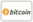Bookcover of A Method Of Lines In The Numerical Solution Of Schrödinger Equation
Booktitle:

# A Method Of Lines In The Numerical Solution Of Schrödinger Equation

LAP LAMBERT Academic Publishing (2012-12-07 )eligible for voucher
ISBN-13:

### 978-3-659-29235-4

ISBN-10:
3659292354
EAN:
9783659292354
Book language:
English
Blurb/Shorttext:
The Method of Lines (MOL) has been one of the simplest but effective technique for solving partial differential equations (PDEs) in which all but one dimension is discretized. This work describes numerical solution of 1-dimensional Schrödinger equation using the method of lines approach (MOL) where spatial dimensions were discretized using some finite difference approximation leaving the time dimension to be the only independent variable in the resulting system of initial value problems. The effect of changing the discretization size on the accuracy of the solution procedure versus changing the step size in the integration of the resulting differential equation was also studied with the incorporation of Simpson’s rule function in MATLAB.
Publishing house:
Website:
https://www.lap-publishing.com/
By (author) :
Number of pages:
144
Published on:
2012-12-07
Stock:
Available
Category:
Other
Price:
59.00 €
Keywords:
Discretization, Finite Difference, Schrodinger Equation, Method of lines (MOL), Nonlinear cubic Schrödinger equation.### Categories